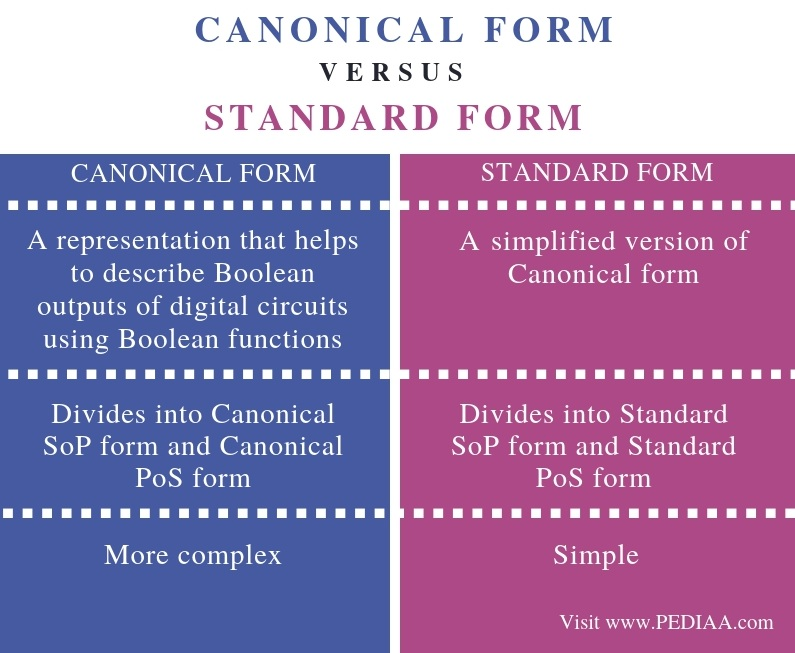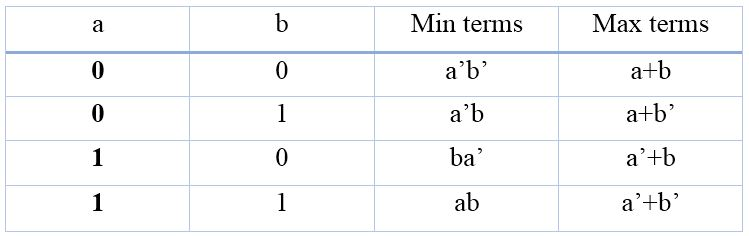# What is the Difference Between Canonical and Standard Form

The main difference between canonical and standard form is that canonical form is a way of representing Boolean outputs of digital circuits using Boolean Algebra while standard form is a simplified version of canonical form that represents Boolean outputs of digital circuits using Boolean Algebra.

Digital circuits operate using digital signals. These signals have discrete binary values: they are zero and one. Zero signifies the false state while one signifies the true state. Boolean algebra is a type of algebra that helps to represent binary numbers and binary variables. Canonical form is a method of representing Boolean functions of Boolean algebra while standard form is a simplified version of canonical form.

### Key Areas Covered

1. What are Min Terms and Max Terms
– Definition, Functionality
2. What is Canonical Form
– Definition, Functionality
3. What is Standard Form
– Definition, Functionality
4. What is the Difference Between Canonical and Standard Form
– Comparison of Key Differences

### Key Terms

Canonical Form, Max Terms, Min Terms, Standard Form## What are Min Terms and Max Terms

For instance, assume that we have two variables as a and b. After performing AND operation, we call the Boolean product terms of these two variables as min terms or standard product terms. These min terms are a’b’, a’b, ba’ and ab.

After performing OR operation, we call the Boolean product terms of these two variables as max terms or standard sum terms. These max terms are a+b, a+b’, a’+b  and a’+b’.

The min terms and max terms of a and b are as follows.## What is Canonical Form

A truth table represents inputs and outputs. If there is ‘n’ number of input variables, then there are 2n number of outputs or combinations or ones and zeros. There are two methods in canonical form to represent an output variable. They are Canonical SoP form and Canonical PoS form.

Canonical SoP stands for Canonical Sum of Products. This form considers themin terms. It is calledsum ofmin terms form. First, it is necessary to recognize the min terms that have 1 as the output variable. After identifying themin terms, the logical OR is used to find the Boolean expression equivalent to the output variable.Figure 1: Digital Circuit

Furthermore, Canonical PoS stands for Canonical Product of Sums. This form concerns the max terms. It is called the Product of Max terms form. First, it is necessary to recognize the max terms that have 0 as the output variable. After identifying the max terms, the logical AND is used to find the Boolean expression equivalent to the output variable.

## What is Standard Form

Another method of representing Boolean outputs is by using standard form. It is divided into two forms as Standard SoP form and Standard PoS form.

The Standard SoP stands for Standard Sum of Products form. It is not necessary for each product term to contain all literals. Therefore, the product terms may or may not be the min terms. There are two steps to follow when obtaining the Standard SoP form of the output variable. The first step is to get the canonical SoP form of the output variable. The second step is to simplify the Boolean function in the Canonical SoP form. If there is more than one output variable, it is possible to follow the same method for other variables. There can be situations where that it is impossible to simplify the canonical SoP form. Then the canonical and standard SoP forms are similar.

The Standard PoS form stands for Standard Product of Sums form. It is not necessary for each product term to contain all literals. Therefore, the sum terms may or may not be the max terms. There are two steps to follow when obtaining the Standard PoS form of the output variable. The first step is to get the canonical PoS form of the output variable. The second step is to simplify the Boolean function in the Canonical PoS form. If there is more than one output variable, it is possible to follow the same method for other variables. There can also be situations where it is impossible to simplify the canonical PoS form. In such cases, canonical and standard PoS forms are similar.

## Difference Between Canonical and Standard Form

### Definition

Canonical form is a representation that helps to describe Boolean outputs of digital circuits using Boolean functions. In contrast, Standard form is a simplified version of Canonical form. These definitions contain the main difference between Canonical and Standard form.

### Division

Another difference between Canonical and Standard form is that Canonical form divides into Canonical SoP form and Canonical PoS form while Standard form divides into Standard SoP form and Standard PoS form.

### Complexity

Moreover, Canonical form is more complex while standard form is simple.

### Conclusion

In brief, Canonical form and standard form are a method of representing Boolean functions of Boolean algebra. The difference between Canonical and Standard form is their complexity as Standard form is a simplified version of Canonical form.

##### Reference:

1. “Canonical Normal Form.” Wikipedia, Wikimedia Foundation, 23 Oct. 2018, Available here.

##### Image Courtesy:

1. “1539113” (CC0) via Maxpixel